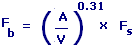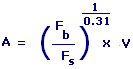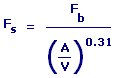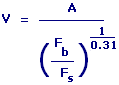Subwoofer Box Enclosure Tuning Frequency Calculators Enter value, select unit and click on calculate. Result will be displayed.

Calculate for Box Enclosure Tuning Frequency
Calculate Air Compliance
Calculate Speaker Resonance Frequency
Calculate Enclosure Volume or for Box

 Web www.calculatoredge.com

Calculate for Box Enclosure Tuning FrequencyFb = Box Enclosure Tuning Frequency A = Air Compliance V = Enclosure Volume or Box Fs = Speaker Resonance Frequency
 Enter your values: Air Compliance: Meter^3 Centimeter^3 Foot^3 Inch^3 Kilometer^3 Yard^3 Liter Milliliter Deciliter Cord Firewood Cord Foot Wood Gallon UK Gallon US Liquid Ounce UK Fluid Ounce US Fluid Quart US Enclosure Volume or Box: Meter^3 Centimeter^3 Foot^3 Inch^3 Kilometer^3 Yard^3 Liter Milliliter Deciliter Cord Firewood Cord Foot Wood Gallon UK Gallon US Liquid Ounce UK Fluid Ounce US Fluid Quart US Speaker Resonance Frequency: Hertz 1 / Second Cycle / Second Rotation / Second Result: Box Enclosure Tuning Frequency: Hertz

Calculate Air ComplianceA = Air Compliance Fb = Box Enclosure Tuning Frequency Fs = Speaker Resonance Frequency V = Enclosure Volume or Box
 Enter your values: Box Enclosure Tuning Frequency: Hertz 1 / Second Cycle / Second Rotation / Second Speaker Resonance Frequency: Hertz 1 / Second Cycle / Second Rotation / Second Enclosure Volume or Box: Meter^3 Centimeter^3 Foot^3 Inch^3 Kilometer^3 Yard^3 Liter Milliliter Deciliter Cord Firewood Cord Foot Wood Gallon UK Gallon US Liquid Ounce UK Fluid Ounce US Fluid Quart US Result: Air Compliance: Meter3

Calculate Speaker Resonance FrequencyFs = Speaker Resonance Frequency Fb = Box Enclosure Tuning Frequency A = Air Compliance V = Enclosure Volume or Box
 Enter your values: Box Enclosure Tuning Frequency: Hertz 1 / Second Cycle / Second Rotation / Second Air Compliance: Meter^3 Centimeter^3 Foot^3 Inch^3 Kilometer^3 Yard^3 Liter Milliliter Deciliter Cord Firewood Cord Foot Wood Gallon UK Gallon US Liquid Ounce UK Fluid Ounce US Fluid Quart US Enclosure Volume or Box: Meter^3 Centimeter^3 Foot^3 Inch^3 Kilometer^3 Yard^3 Liter Milliliter Deciliter Cord Firewood Cord Foot Wood Gallon UK Gallon US Liquid Ounce UK Fluid Ounce US Fluid Quart US Result: Speaker Resonance Frequency: Hertz

Calculate Enclosure Volume or for BoxV = Enclosure Volume or Box A = Air Compliance Fs = Speaker Resonance Frequency Fb = Box Enclosure Tuning Frequency
 Enter your values: Air Compliance: Meter^3 Centimeter^3 Foot^3 Inch^3 Kilometer^3 Yard^3 Liter Milliliter Deciliter Cord Firewood Cord Foot Wood Gallon UK Gallon US Liquid Ounce UK Fluid Ounce US Fluid Quart US Box Enclosure Tuning Frequency: Hertz 1 / Second Cycle / Second Rotation / Second Speaker Resonance Frequency: Hertz 1 / Second Cycle / Second Rotation / Second Result: Enclosure Volume or Box: Meter3LINKSDISCLAIMERCONTACT US